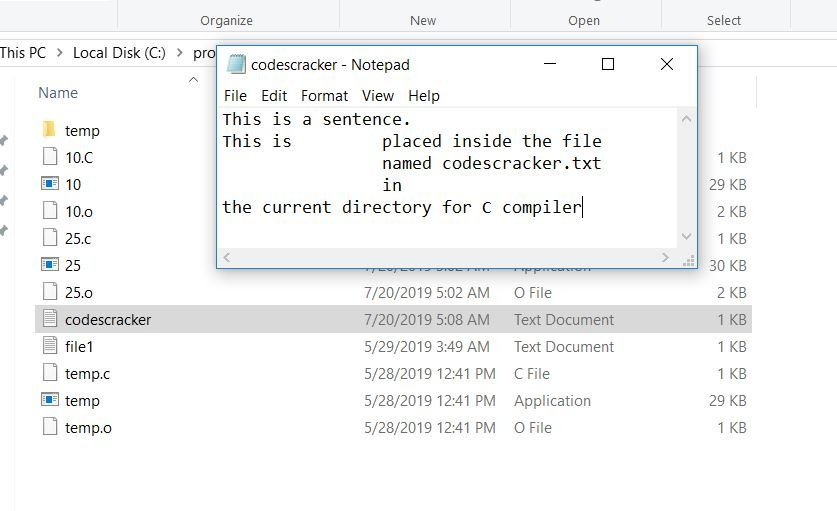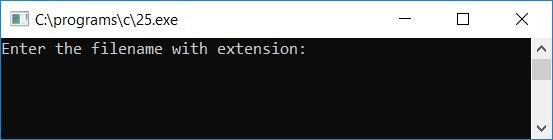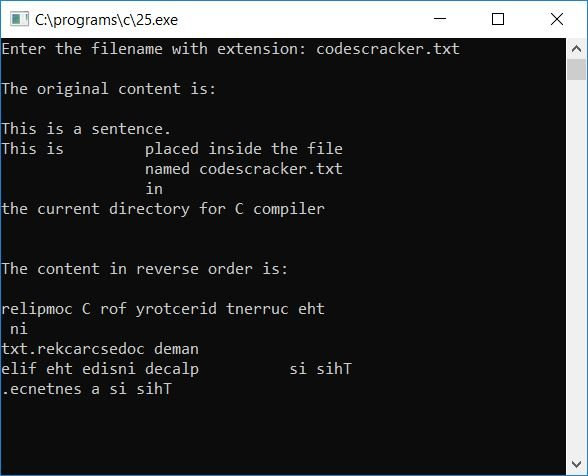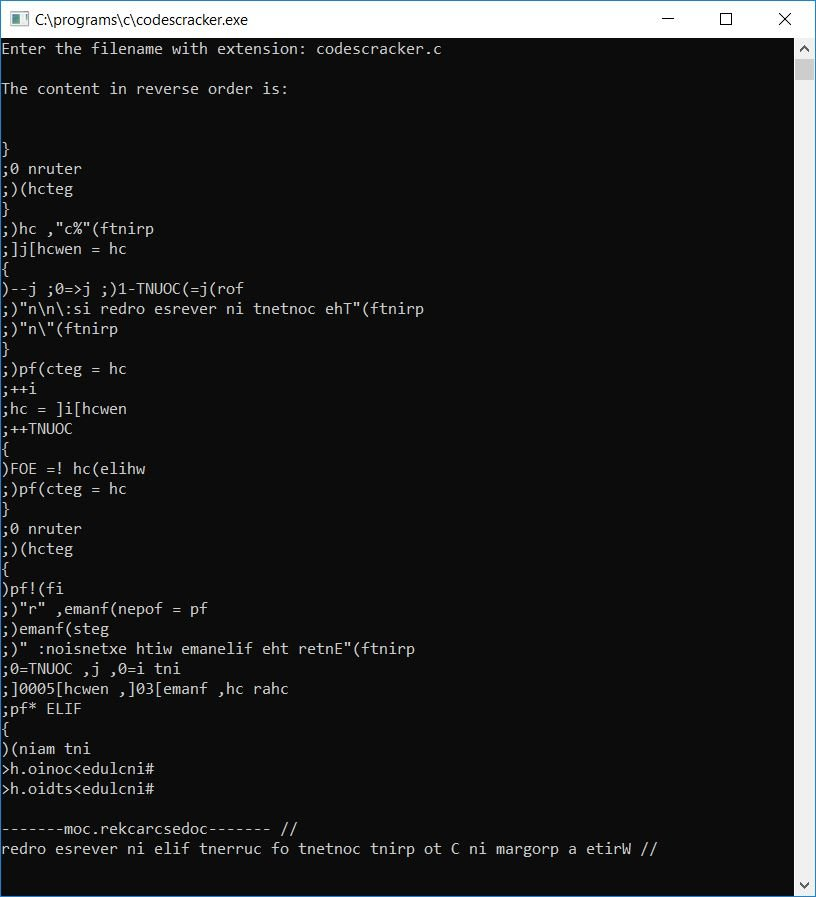# C Program to Print the Content of a File in Reverse Order

In this article, we will learn how to create a program in C that prints the content of any given file (given by the user at run-time) in reverse order.

### Things to Do Before the Program

Before creating the program that prints any file's content in reverse order, Let's first create a file with some content inside it. For example, let's write the following content in any text editor, say NotePad:

```This is a sentence.
This is		placed inside the file
named codescracker.txt
in
the current directory for C compiler```

In the current directory, save the file with the name codescracker.txt. Here is the screenshot of the file along with its content and the folder where the file codescracker.txt is created and saved:## Print File Content in Reverse Order

The question is: write a program in C that displays the content of a file in reverse order. The filename should be entered at run-time. The answer to this question is:

```#include<stdio.h>
#include<conio.h>
int main()
{
FILE *fp;
char ch, fname, newch;
int i=0, j, COUNT=0;
printf("Enter the filename with extension: ");
gets(fname);
fp = fopen(fname, "r");
if(!fp)
{
printf("Error in opening the file...\nExiting...");
getch();
return 0;
}
printf("\nThe original content is:\n\n");
ch = getc(fp);
while(ch != EOF)
{
COUNT++;
putchar(ch);
newch[i] = ch;
i++;
ch = getc(fp);
}
printf("\n\n\n");
printf("The content in reverse order is:\n\n");
for(j=(COUNT-1); j>=0; j--)
{
ch = newch[j];
printf("%c", ch);
}
printf("\n");
getch();
return 0;
}```

The above program was written in the Code::Blocks IDE; therefore, after a successful build and run, following is the sample run.

This program will ask the user to enter any filename along with its extension that is present inside the current directory (where the above source code is saved). We've already created a file called codescracker.txt in the current directory.Therefore, we can operate on that file for practical purposes to see how the output of the above program works:Now supply the name of the file that was created earlier, say codescracker.txt, and press the ENTER key to see the content in its original order first and then the same content in reverse order at the end of the output, as shown here:#### Program Explained

• Receive the name of the file along with its extension.
• Now open the file using the "fopen()" function and initialize it to any "FILE" pointer, say "fp."
• The function "fopen()" accepts two arguments: the name of the file with its extension (that will be operated on) and the mode in which the file will be opened.
• If the file does not exist or there is any error while opening the file, print the error message and exit from the program.
• Otherwise, if the file is successfully opened, proceed to the next step in the file's operation, which is to print its content in reverse order.
• First, print the file's content in its original order.
• To do this, get the first character of the file using the "fgetc()" function, and initialize the first character to any variable, say "ch." Here the function "fgetc()" takes one argument that will be a file pointer, say "fp." Here "fp" is the file pointer of the filename entered by the user at run-time, say "codescracker.txt."
• Whenever you call "fp," the content of a file named "codescracker.txt" gets called using the pointer.
• Create a "while" loop that runs until "End-Of-File" (EOF) gets reached.
• That is, we have to apply the "while" loop's condition statement as "ch!= EOF." It states that the loop gets run until the value of "ch" gets equal to "EOF." The value of EOF is "-1."
• EOF indicates that there is nothing left inside the file, or no content left inside the file.
• Inside the "while" loop, we have a variable called "COUNT" (initialized with 0 at the start of the program) that counts the total number of characters present inside the file.
• Use the "putchar()" function to put the character on the output screen. That is, "putchar (ch)" puts the value of "ch" on the output screen.
• Now initialize the value of "ch" in an array, saying "newch[]". The indexing of this array must start with 0, therefore we have already initialized a variable, say "i," with 0 at the start of the program, and therefore we have used the "newch[i] = ch;" statement for the first time inside the "while" loop's first run. That is, at the first run of the loop, "ch" gets initialized "newch" and at the second run, a new value of "ch" gets initialized to "newch" and so on. Therefore, we have incremented the value of "i" after initializing the value of "ch" to initialize the next value of "ch" at the next index of the array.
• Never forget to use the "getc()" function to get the next character. Therefore, we have initialized "getc(fp)" to "ch" at the end of the loop.
• In this way, we have printed the content of the file (as it is) in its original order.
• Now create another loop ("for" loop this time) to print the content of the file in reverse order.
• Because we have an array named "newch[]" that contains all of the characters of the file (characters of the file means file content). And we have a variable named "COUNT" that holds the length of the file's content, or the total number of characters present inside the given file.
• Now to print the content of the file in reverse order, just create a "for" loop that runs from "COUNT-1" to "0."
• We have started the loop with the last index of the array.
• That is, we have printed the last character at first and the first character at last to print the file content in reverse order.
• For example, if the content of the file is "This is codescracker,"  then here is the list of step-by-step dry runs of the "while" loop. Here the value of "COUNT" gets incremented each time when the program flow goes inside the loop, a character or value of "ch" gets printed each time, a character gets initialized one by one to the array named "newch[]," the value of variable "i" gets incremented each time, and the next character gets scanned using the "ch = getc(fp);" statement as shown in the list of dry runs given below. We have scanned the first character of the file's content using the "ch = getc(fp);" statement before going to the "while" loop, therefore "t" gets initialized to "ch" before the first run of the "while" loop:
• At the first run of the "while" loop:
The value of "COUNT" becomes 1.
"t" gets printed on the output.
"t" gets initialized to "newch"
The value of "i" becomes 1.
"h" gets initialized to "ch."
• At the second run of the "while" loop:
The value of "COUNT" becomes 2.
"h" gets printed on output.
"h" gets initialized to "newch"
The value of "i" becomes 2.
"i" gets initialized to "ch."
• At the third run of the "while" loop:
The value of "COUNT" becomes 3.
"i" gets printed on the output.
"i" gets initialized to "newch"
The value of "i" becomes 3.
"s" gets initialized to "ch."
• :
• At twentieth run of the "while" loop:
The value of "COUNT" becomes 20.
"r" gets printed on the output.
"r" gets initialized to "newch"
The value of "i" becomes 20.
EOF (-1) gets initialized to "ch."
• Therefore, to print the content of the file in reverse order, we have to print the array's content starting from the last index.
• That is, "COUNT-1" or "20-1" or "19" gets initialized to "j" (the loop variable), as "19" is greater than or equal to 0, therefore program flow goes inside the "for" loop, and "newch[j]" or "newch" or "r" gets initialized to "ch." And the value of "ch" gets printed.
• In this way, all the content of the file gets printed in reverse order.

## Print the contents of the current file in reverse order

If you want to print the content of the current file (to which you are writing the current program, say codescracker.c), then we have to increase the size of the array, say newch[], because the code given above may be more than 500 characters. Therefore, increase the size and make it to 5000, which is newch.

Note: Never forget to save the file before running it and enter the current file name to print its content in reverse order.

Let me create a program for you to check for the current file. This program will ask the user to enter the file name and extension and will print the file in reverse order. You can enter any filename with an extension. However, we will look for the current file here.

```#include<stdio.h>
#include<conio.h>
int main()
{
FILE *fp;
char ch, fname, newch;
int i=0, j, COUNT=0;
printf("Enter the filename with extension: ");
gets(fname);
fp = fopen(fname, "r");
if(!fp)
{
getch();
return 0;
}
ch = getc(fp);
while(ch != EOF)
{
COUNT++;
newch[i] = ch;
i++;
ch = getc(fp);
}
printf("\n");
printf("The content in reverse order is:\n\n");
for(j=(COUNT-1); j>=0; j--)
{
ch = newch[j];
printf("%c", ch);
}
getch();
return 0;
}```

Here is the final snapshot of the sample run:C Quiz

« Previous Program Next Program »